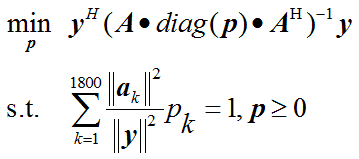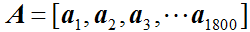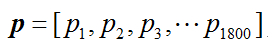# Can cvx solve this problem?Everything in the objective function other than p is input data.
y and a is 10 * 1 vector.
A is 10 * 1800 array.1 Like

help matrix_frac

`````` matrix_frac   Matrix fractional function.
matrix_frac(x,Y), where Y is a square matrix and x is a vector of the
same size, computes x'*(inv(Y)*x) if Y is Hermitian positive definite, and
+Inf otherwise.

An error results if Y is not a square matrix, or the size of
the vector x does not match the size of matrix Y.

Disciplined convex programming information:
matrix_frac is convex and nonmonotonic (at least with respect to
elementwise comparison), so its argument must be affine.``````